Courses

# Test: Soil Mechanics- 7

## 10 Questions MCQ Test GATE Civil Engineering (CE) 2022 Mock Test Series | Test: Soil Mechanics- 7

Description
This mock test of Test: Soil Mechanics- 7 for Civil Engineering (CE) helps you for every Civil Engineering (CE) entrance exam. This contains 10 Multiple Choice Questions for Civil Engineering (CE) Test: Soil Mechanics- 7 (mcq) to study with solutions a complete question bank. The solved questions answers in this Test: Soil Mechanics- 7 quiz give you a good mix of easy questions and tough questions. Civil Engineering (CE) students definitely take this Test: Soil Mechanics- 7 exercise for a better result in the exam. You can find other Test: Soil Mechanics- 7 extra questions, long questions & short questions for Civil Engineering (CE) on EduRev as well by searching above.
QUESTION: 1

### Which of the following assumptions is wrongly stated in regard to Rankine’s theory of earth pressure?

Solution:

The Rankine's theory assumes that there is no wall friction, the ground and failure surfaces are straight planes, and that the resultant force acts parallel to the backfill slope.

The soil mass is homogeneous and semi-infinite and retaining wall yields about the base.

In case of retaining structures, the earth retained may be filled up earth or natural soil. These backfill materials may exert certain lateral pressure on the wall.

QUESTION: 2

### A retaining wall of height H with smooth vertical backface supports a backfill inclined at an angle β with the horizontal. The backfill consists of cohesionless soil having an angle of internal friction ϕ. If the active lateral thrust acting on the wall is Pa, which one of the following statements is TRUE?

Solution: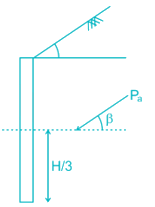For the retaining with supporting backfill of cohesionless soil having an angle of internal friction ϕ and inclined at an angle β with the horizontal. Active Earth Pressure (Pa) acts at a height H/3 from the base of the wall and at an angle β with the horizontal

QUESTION: 3

### Consider the following statements: 1. Method of slices overestimates the value of factor of safety. 2. The exact value of factor of safety is obtained in ϕT = 0 analysis. 3. Reduction in shearing resistance in embankment is caused by tension crack at the top of it. 4. The plane surface of failure never occurs in saturated soils. Which of the statements given above are correct?

Solution:

The actual shape of a slip surface in the case of finite slopes is curvilinear. The assumption of a circular slip surface and its application for stability analysis was developed in Sweden. The method is known as the Swedish circle method or method of slices. This method neglects the effect of forces acting on the sides of the vertical strips. The error due to this is on safe side. i.e. the factor of safety obtained is generally less than that obtained from more accurate methods such as Bishop’s method which also consider the forces on the sides of vertical strips.

ϕT = 0 means that friction is not mobilized. In the actual case the resistance to shear will be due to friction and cohesion both thus exact value of factor of safety cannot be obtained for ϕT = 0.

QUESTION: 4

What is active earth pressure at the bottom of a vertical cut, 4.0 m deep in soil with ϕ’ = 30° and c’ = 20 kN/m2 and γ = 25 kN/m3?

Solution: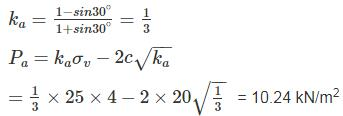QUESTION: 5

A 5m high vertical earth retaining wall having smooth vertical back retains non-cohesive soil with angle of internal friction 35° and unit weight γ = 18 kN/m3. If the wall is free to field and the water table is at a depth of 2m below the top of the wall, then the total horizontal active thrust (kN) per unit length acting on the wall will be

Take γsat = 20 kN/m3, γω = 10kN/m3.

Solution: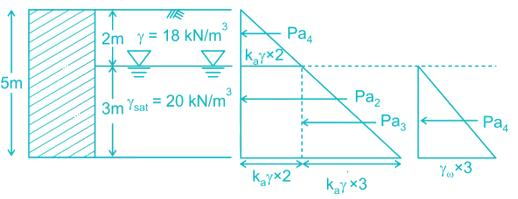Total active earth pressure at 2m below the Ground level = kaγ×2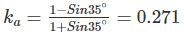Active thrust:
Pa1 = 12 × ka × γ × 2 × 2 =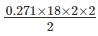Pa1 = 9.756 kN/m
Total active earth pressure at 5m below the Ground level:

= ka × γ ×2 + kaγ1×3 + γω × 3

= 0.271 × 18 × 2 + 0.271 × [20 – 10] × 3 + 10 × 3

= 47.88 kN/m2

Active thrust:-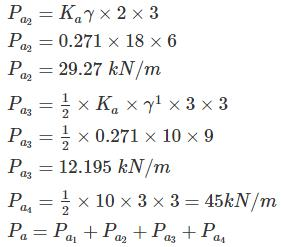Pa = 9.756 + 29.27 + 12.195 + 45

Pa = 96.221 kN/m

*Answer can only contain numeric values
QUESTION: 6

A cutting is to be made in clay having cohesion 50 kPa and unit weight 22 kN/m3. If the maximum depth of cut is limited to 8.30m, determine the stability Factor against the factor of safety w.r.t. cohesion equal to 2.

Solution:

Concept: Stability Number is given by: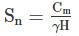Cm = mobilised cohesion

γ = unit weight of the soil

H = Depth of vertical cut

Sn = Stability No.

Factor of Safety w.r.t. cohesion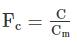C = Cohesion of soil

Stability factor = 1/ Stability Number

Calculation:

C = 50 kN/m2

γ = 22 kN/m3

H = 8.30 m

Fc = 2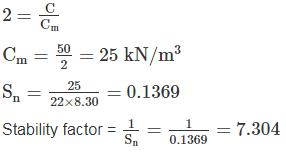*Answer can only contain numeric values
QUESTION: 7

A granular soil has a saturated unit weight of 20 kN/m3 and an effective angle of shearing resistance of 30°. The unit weight of water is 9.81 kN/m3. A slope is to be made on this soil deposit in which the seepage occurs parallel to the slope up to the free surface. Under this seepage condition for a factor of safety of 1.5, the safe slope angle (in degree, round off to 1 decimal place) would be ______.

Solution: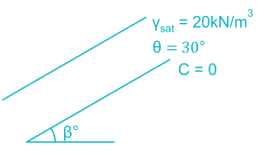FOS = 1.5

If there is seepage parallel to the slope

β = 11.095°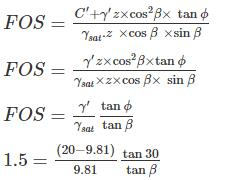*Answer can only contain numeric values
QUESTION: 8

The soil profile above the bedrock surface at 30° infinite slope is shown in the figure where Su is undrained shear stress and y is total unit weight. The slip will occur at depth of __m. Provide an answer up to 2 decimal places.

Solution:

The slip will occur when shear stress is greater than shear strength.

Assumption 1

z1 ≤ 4m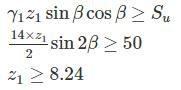Hence assumption wrong.

Assumption 2

Z > 4m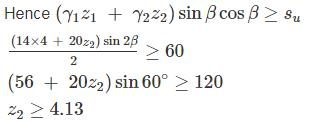Hence depth of slip = 4 + 4.13 = 8.13 m

*Answer can only contain numeric values
QUESTION: 9

Three soil profile is used as backfill behind a smooth vertical retaining wall as shown in the figure below. The ratio of total active earth pressure (kN/m2) at Point P to Point Q will be _____ (up to two decimal places)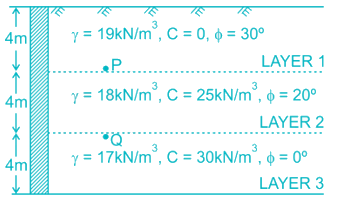Solution:

Active earth Pressure coefficient (ka)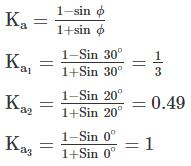Active earth Pressure at Point P is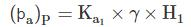H1 = 4m

γ1 = 19 kN/m3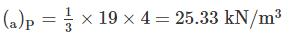Active earth Pressure at Point Q is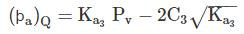PV = total vertical pressure at point Q

PV = 19 × 4 + 18 × 4 = 148 kN/m2

Ka3=1

C3 = 30 kN/m3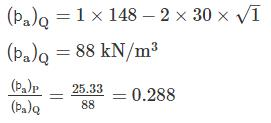*Answer can only contain numeric values
QUESTION: 10

A 5 m high retaining wall is as shown in figure determine ranking active earth pressure (in kN/m) per unit length of the retaining wall after the formation of tension crack: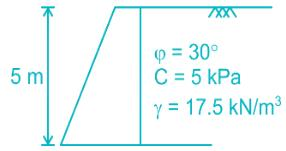Solution: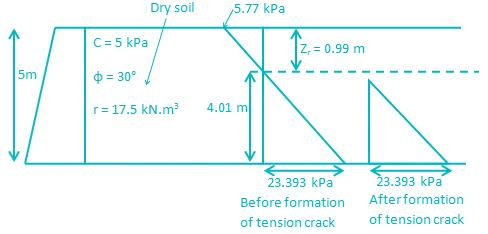Ka = (1 – sin φ)/ (1 + sin φ) = 0.33

At z = 0, σ’v = 0

pa = σv ka – 2c √ka = – 2 × 5 × √0.33

= – 5.77 kPa

Depth of tension crack

(zv) = 2c/(r √ka) = (2 × 5)/(17.5 × √0.33) = 0.99m

At bottom,

σ'v = (17.55 x 5) = 87.5 kPa

Pa = 87.5 × 0.33 – 5.77 = 23.392 kPa

After formation of tension crack = 1/2 × 23.393 × 4.01 = 46.9 kN/m